Next: Calculus of Variations Up: Classical Mechanics Previous: Lagrange's Equation

# Generalized Momenta

Consider the motion of a single particle moving in one dimension. The kinetic energy is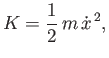(B.23)

where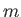is the mass of the particle, andits displacement. The particle's linear momentum is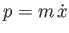. However, this can also be written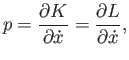(B.24)

because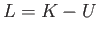, and the potential energy,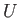, is independent of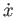.

Consider a dynamical system described by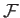generalized coordinates,, for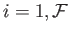. By analogy with the previous expression, we can define generalized momenta of the form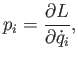(B.25)

for. Here,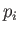is sometimes called the momentum conjugate to the coordinate. Hence, Lagrange's equation, (B.22), can be written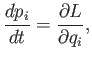(B.26)

for. Note that a generalized momentum does not necessarily have the dimensions of linear momentum.

Suppose that the Lagrangian,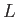, does not depend explicitly on some coordinate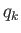. It follows from Equation (B.26) that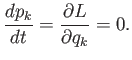(B.27)

Hence,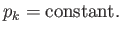(B.28)

The coordinateis said to be ignorable in this case. Thus, we conclude that the generalized momentum associated with an ignorable coordinate is a constant of the motion.Next: Calculus of Variations Up: Classical Mechanics Previous: Lagrange's Equation
Richard Fitzpatrick 2016-01-25